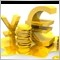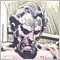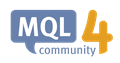# Using iCustom with Indicator that has several inputs12613

Hi all.

I am trying to extract values from an indicator (assume the name is "ABC_Indicator") that has several input values. I have source code of the indicator.

Hence I use the iCustom function to create the following function to extract the buffer values ,as follows.

``` double  GetEntrySignalBuffers(int buffer)
{
return ( iCustom (

Symbol(),
Period(),
"ABC_Indicator",//File Path of indicator
// custom indicator input parameters (if necessary)
buffer,//Index Buffer
0
));
}```

That is the default version of the iCustom assuming I am extracting the buffer values with the default values of the indicator .  I checked and the values are correct.

My question is when I want to introduce in my above function the optional inputs of "ABC_Indicator" , is there a way to know which ones of the "ABC_Indicator" inputs and in which order I should include in the iCustom function to get the correct result.

Thank you4836

Michalis Phylactou:

Hi all.

I am trying to extract values from an indicator (assume the name is "ABC_Indicator") that has several input values. I have source code of the indicator.

Hence I use the iCustom function to create the following function to extract the buffer values ,as follows.

That is the default version of the iCustom assuming I am extracting the buffer values with the default values of the indicator .  I checked and the values are correct.

My question is when I want to introduce in my above function the optional inputs of "ABC_Indicator" , is there a way to know which ones of the "ABC_Indicator" inputs and in which order I should include in the iCustom function to get the correct result.

Thank you

I typically include all of the inputs from the indicator in the order they are declared in the indicator.

According to the documentation:

"The passed parameters and their order must correspond with the declaration order and the type of extern variables of the custom indicator. If the values of input parameters  is not specified, the default values will be used."Functions - Language Basics - MQL4 Reference
• docs.mql4.com
Every task can be divided into subtasks, each of which can either be directly represented in the form of a code, or divided into smaller sub-tasks. This method is called of the function definition. The function header includes a description of the return value type, name (identifier) and formal parameters.  The number of parameters passed to...23729

Michalis Phylactou: My question is when I want to introduce in my above function the optional inputs of "ABC_Indicator" , is there a way to know which ones of the "ABC_Indicator" inputs and in which order I should include in the iCustom function to get the correct result.
You add parameters in the correct order, correct type starting with the first. You can't skip any, except the last ones (defaults).